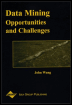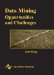# Index ZList of Figures Data Mining: Opportunities and Challenges by John Wang (ed) Idea Group Publishing 2003Brought to you by Team-Fly

## Chapter I: A Survey of Bayesian Data Mining

Figure 1: Graphical models, dependence or independence?
Figure 2: Graphical models, conditional independence?
Figure 3: Symptoms and causes relevant to heart problem.
Figure 4: Graphical models detecting co-variation.
Figure 5: Association between age and posterior superior vermis volume depends on diagnosis. The principal directions of variation for controls(o) and affected subjects (+) are shown.

## Chapter II: Control of Inductive Bias in Supervised Learning Using Evolutionary Computation-A Wrapper-Based Approach

Figure 1: A composite learning framework.
Figure 2: Systems for attribute-driven unsupervised learning and model selection.
Figure 3: Mean classification accuracy of specialists vs. moderators for all (52) partitions of 5-attribute modular parity problem.
Figure 4: Phased autocorrelogram (plot of autocorrelation shifted over time) for crop condition (average quantized estimates).

## Chapter III: Cooperative Learning and Virtual Reality-Based Visualization for Data Mining

Figure 1: Data preprocessing and data mining tasks [Adapted from Docherty & Beck, 2001].
Figure 2: Graphical representation of rules.
Figure 3: Rule Builder interface.

## Chapter IV: Feature Selection in Data Mining

Figure 1: ELSA pseudo-code.
Figure 2: The ELSA/ANN model.
Figure 3: Lift curves of three models that maximize the hit rate when targeting the top 20% of prospects.
Figure 4: Lift curves of three models that maximize the area under lift curve when targeting up to top 50% of prospects.
Figure 5: The pseudo-code of ELSA/EM.
Figure 6: A few two-dimensional projections of the synthetic data set.
Figure 7: The candidate fronts of ELSA/EM model.
Figure 8: Candidate fronts for K = 5 based on Faccuracy evolved in ELSA/EM. It is captured at every 3,000 solution evaluations and two fronts (t = 18,000 and t = 24,000) are omitted because they have the same shape as the ones at t = 15,000 and t = 21,000, respectively.
Figure 9: Estimated survival curves for the three groups found by ELSA/EM.
Figure 10: Pseudo-code of Meta-Evolutionary Ensembles (MEE) algorithm.
Figure 11: Graphical depiction of energy allocation in the MEE. Individual classifiers (small boxes in the environment) receive energy by correctly classifying test points. Energy for each ensemble is replenished between generations based on the accuracy of the ensemble. Ensembles with higher accuracy have their energy bins replenished with more energy per classifier, as indicated by the varying widths of the bins.
Figure 12: The relationship between the predictive accuracy and ensemble size (left), and between the predictive accuracy and ensemble diversity (right) with 95% confidence interval on the Soybean data. We observed similar patterns on other data sets.

## Chapter V: Parallel and Distributed Data Mining through Parallel Skeletons and Distributed Objects

Figure 1: Some of the parallel skeletons available in SkIE, their graphical representation and concrete syntax. Examples of simple data types inside interface definitions, in place of full parameter lists.
Figure 2: Different representations for boolean transaction data. The lattice of itemsets, with the subsets of frequent set ABD put in evidence.
Figure 3: Apriori pseudo-code for frequent itemsets.
Figure 4: Pseudo-code of partitioned ARM with mapping to parallel modules.
Figure 5: SkIE code for the parallel APriori.
Figure 6: Parallel structure of Partitioned Apriori.
Figure 7: Example of decision tree.
Figure 8: Pseudocode of C4.5, tree-building phase.
Figure 9: SkIE code for parallel C4.5.
Figure 10: Block Structure of parallel C4.5.
Figure 11: Pseudo-code of DBSCAN.
Figure 12: Parallel decomposition of DBSCAN.
Figure 13: The SkIE code of parallel DBSCAN.
Figure 14: Block structure of parallel DBSCAN.
Figure 15: Average number of points per query answer, versus parallelism and epsilon.
Figure 16: Apriori efficiency versus parallelism (* = centralized I/O).
Figure 17: Apriori speedup versus parallelism.
Figure 18: Parallel Apriori, T3E completion time.

## Chapter VI: Data Mining Based on Rough Sets

Figure 1: Rule set.
Figure 2: Rule set.
Figure 3: Rule set computed by LEM1 from consistent data.
Figure 4: Rule set computed by LEM1 from inconsistent data.
Figure 5: Rule set computed by LEM 2.2, 2, 2.
Figure 6: Rule set computed by All Global Coverings option of LERS.
Figure 7: Rule set computed by All Rules option of LERS.

## Chapter VIII: Mining Text Documents for Thematic Hierarchies Using Self-Organizing Maps

Figure 1: The formation of neurons in the map.
Figure 2: (a) A two-level hierarchy comprises a super-cluster as root node and several clusters as child nodes. (b) The dominating neuron k is selected and used as a super-cluster. Its neighboring neurons compose the super-cluster. We only show a possible construction of the hierarchy here.
Figure 3: The text categorization process.
Figure 4: The category hierarchies of CORPUS-1.
Figure 5: English translation of Figure 4.
Figure 6: One of the category hierarchies developed from CORPUS-2.

## Chapter XI: Bayesian Data Mining and Knowledge Discovery

Figure 1: Graph (causal) model of a BBN.
Figure 2: Graphical model of a fictitious medical BBN.

## Chapter XII: Mining Free Text for Structure

Figure 1: How FAQ Finder works.
Figure 2: A sample from a FAQ about caffeine.
Figure 3: Criss-crossing sequences.
Figure 4: Stable marker structure.
Figure 5: Sample text.
Figure 6: Layout of Figure 5.
Figure 7: Logical map of Figure 5.
Figure 8: FAQ Minder's architecture.
Figure 9: Layout change.

## Chapter XIII: Query-By-Structure Approach for the Web

Figure 1: CLaP system architecture.
Figure 2: Visual Interface module.
Figure 3: Example of an SQL query.
Figure 4: Neural network query-by-structure process.

## Chapter XIV: Financial Benchmarking Using Self-Organizing Maps-Studying the International Pulp and Paper Industry

Figure 1: (a) Rectangular lattice (size 4 4), and (b) Hexagonal lattice (size 4 4).
Figure 2: (a) A randomly initialized network after one learning step and (b) a fully trained network (Source: Kohonen, 1997).
Figure 3: (a) Operating Margin, (b) Return on Equity, and (c) Equity to Capital feature planes.
Figure 4: (a) The final U-matrix map, and (b) identified clusters on the map.
Figure 5: Country averages for the years 1995-2000.
Figure 6: Market pulp prices 1985-99 (Source: Mets teollisuus ry Internal report; Keaton, 1999).
Figure 7: Movements of the top five pulp and paper companies during the years 1995-2000.
Figure 8: Japanese companies during the years 1997-2000.
Figure 9: The best companies.
Figure 10: The poorest performing companies.

## Chapter XV: Data Mining in Health Care Applications

Figure 1: Cooperative CHIN Implementation Model.
Figure 2: Market/location matrix.
Figure 3: The SAS Enterprise data-mining technology (http://www.sas.com/products/miner/index.html).

## Chapter XVII: Data Mining in Information Technology and Banking Performance

Figure 1: Efficient frontier.
Figure 2: IT impact on banking performance.

## Chapter XVIII: Social, Ethical and Legal Issues of Data Mining

Figure 1: Ethical violations often fuel the creation of laws and regulations.
Figure 2: The line dividing public and private consumer information continues to shift.

## Chapter XIX: Data Mining in Designing an Agent-Based DSS

Figure 1: Multiagent architecture for the dynamic DSS.
 Brought to you by Team-FlyData Mining: Opportunities and Challenges
ISBN: 1591400511
EAN: 2147483647
Year: 2003
Pages: 194
Authors: John Wang